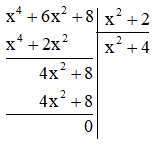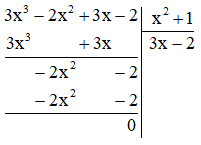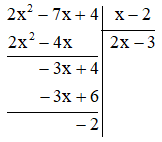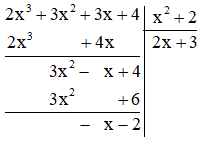## SBT Prize at the end of chapter 7 – Math 7 SBT Horizon – Math Book

SBT Prize End of Chapter 7 – Math 7 SBT Horizon
==========

### Solution 1 page 33 SBT Math 7 Creative horizon episode 2 – CTST

Let $$B = x{y^3} + 4xy – 2{x^2} + 3$$. Calculate the value of the expression $$B$$ when $$x = – 1$$, $$y = 2$$.

Detailed instructions for solving Lesson 1

Solution method

Substitute the values ​​of the variable and calculate.

Detailed explanation

Substituting $$x = – 1$$, $$y = 2$$ into $$B = x{y^3} + 4xy – 2{x^2} + 3$$ we have

$$B = \left( { – 1} \right){.2^3} + 4\left( { – 1} \right).2 – 2. {\left( { – 1} \right)^2 } + 3 = – 15$$.

–>

— *****

### Solution 2 page 33 SBT Math 7 Creative horizon episode 2 – CTST

Which of the following expressions is a monomial of one variable?

a) $$2y$$;

b) $$3x + 5$$;

c) $$12$$;

d) $$\frac{1}{3}{t^2}$$.

Detailed instructions for solving Lesson 2

Solution method

A monomial of a variable is an algebraic expression consisting of only a number, or a variable, or a product between numbers and the variable.

Detailed explanation

The one-variable monomial is a; c; d.

–>

— *****

### Solution 3 page 33 SBT Math 7 Creative horizon episode 2 – CTST

Which of the following expressions is a one-variable polynomial?

$$5 – 2x$$; $$6{x^2} + 8{x^3} + 3x – 2$$;

$$\frac{2}{{x – 1}}$$; $$\frac{1}{4}t – 5$$.

Detailed instructions for solving Lesson 3

Solution method

Understand the concept of one-variable monomial, one-variable polynomial to determine.

A monomial of a variable is an algebraic expression consisting of only a number, or a variable, or a product between numbers and the variable.

A one-variable polynomial is the sum of all mononomials of the same variable.

Detailed explanation

The one-variable polynomials are “ $$5 – 2x;\,\,6{x^2} + 8{x^3} + 3x – 2$$; $$\frac{1}{4}t – 5$$.

–>

— *****

### Solution 4 page 33 SBT Math 7 Creative horizon episode 2 – CTST

Write a quaternary one-variable polynomial with 5 terms.

Detailed instructions for solving Lesson 4

Solution method

A quaternary one-variable polynomial means that the degree of the largest variable is 4.

Detailed explanation

A quaternary one-variable polynomial with 5 terms is:

A(x) = x4 – 2x3 + 3x2 – 4x + 5.

Comment: This post has many answers.

–>

— *****

### Solve problem 5 page 33 SBT Math 7 Creative horizon episode 2 – CTST

State the degrees of the following polynomials:

$$A = 5{x^2} – 2{x^4} + 7$$;

$$B = 17$$;

$$C = 3x – 4{x^3} + 2{x^2} + 1$$.

Detailed instructions for solving Lesson 5

Solution method

The degree of the polynomial is the maximum exponent of the variable after reducing the polynomial.

Detailed explanation

The degree of $$A = 5{x^2} – 2{x^4} + 7$$ is 2.

The degree of $$B = 17$$ is 0.

The degree of $$C = 3x – 4{x^3} + 2{x^2} + 1$$ is 3.

–>

— *****

### Solution 6 page 33 SBT Math 7 Creative horizon episode 2 – CTST

Let the polynomial $$P\left( x \right) = {x^3} + 64$$. Find the root of the polynomial in the set $$\left\{ {0;4; – 4} \right\}$$

Detailed instructions for solving Lesson 6

Solution method

Replace $$x = {x_0}$$ into $$P\left( x \right)$$ if $$P\left( {{x_0}} \right) = 0$$ then $$x = {x_0}$$ is the solution of $$P\left( x \right)$$.

Detailed explanation

With $$x = 0$$ we have $$P\left( 0 \right) = {0^3} + 64 = 64 \ne 0$$, so $$0$$ is not a solution of $$P \left( x \right)$$.

With $$x = 4$$ we have $$P\left( 4 \right) = {4^3} + 64 = 128 \ne 0$$, so $$4$$ is not a solution of $$P \left( x \right)$$.

With $$x = – 4$$ we have $$P\left( { – 4} \right) = {\left( { – 4} \right)^3} + 64 = 0$$, deduce $$– 4$$ is the solution of $$P\left( x \right)$$.

So $$– 4$$ is the solution of $$P\left( x \right)$$.

–>

— *****

### Solution 7 page 33 SBT Math 7 Creative horizon episode 2 – CTST

The length of the triangle is $$3y + 2$$; $$6y – 4$$ and the perimeter is $$23y – 5$$. Find the unknown side in that triangle.

Detailed instructions for solving Lesson 7

Solution method

The perimeter of a triangle is equal to the sum of its three sides. So, if we want to find the unknown edge, we subtract the known edges from the perimeter.

Detailed explanation

The unknown side in that triangle is $$23y – 5 – \left( {3y + 2} \right) – \left( {6y – 4} \right) = 14y – 3$$.

–>

— *****

### Solve problem 8 page 34 SBT Math 7 Creative horizon episode 2 – CTST

Let the polynomial $$M\left( x \right) = 3{x^5} – 4{x^3} + 9x + 2$$. Find the polynomials $$N\left( x \right)$$, $$Q\left( x \right)$$ such that $$N\left( x \right) – M\left( x \right) = – 5{x^4} – 4{x^3} + 2{x^2} + 8x$$ and $$Q\left( x \right) + M\left( x \right) = 3{x ^4} – 2{x^3} + 9{x^2} – 7$$

Detailed instructions for solving Lesson 8

Solution method

Step 1: Add and subtract mononomials of the same variable to reduce the given polynomial.

Step 2: Sort the monomials by the descending power of the variable.

Step 3: Perform the calculation horizontally or vertically.

Detailed explanation

$$N\left( x \right) – M\left( x \right) = – 5{x^4} – 4{x^3} + 2{x^2} + 8x \Rightarrow N\left( x \right) = – 5{x^4} – 4{x^3} + 2{x^2} + 8x + M\left( x \right)$$

$$– 5{x^4} – 4{x^3} + 2{x^2} + 8x + 3{x^5} – 4{x^3} + 9x + 2 = 3{x^5} – 5{x^4} – 8{x^3} + 2{x^2} + 17x + 2$$

So $$N\left( x \right) = 3{x^5} – 5{x^4} – 8{x^3} + 2{x^2} + 17x + 2$$.

$$Q\left( x \right) + M\left( x \right) = 3{x^4} – 2{x^3} + 9{x^2} – 7 \Rightarrow Q\left( x \ right) = 3{x^4} – 2{x^3} + 9{x^2} – 7 – M\left( x \right)$$

$$3{x^4} – 2{x^3} + 9{x^2} – 7 – \left( {3{x^5} – 4{x^3} + 9x + 2} \right) = 3{x^4} – 2{x^3} + 9{x^2} – 7 – 3{x^5} + 4{x^3} – 9x – 2 = – 3{x^5} + 3{x^4} + 2{x^3} + 9{x^2} – 9x – 9$$

–>

— *****

### Solve lesson 9 page 34 SBT Math 7 Creative horizon episode 2 – CTST

Perform multiplication.

a) $$\left( {4x – 5} \right)\left( {3x + 4} \right)$$;

b) $$\left( {2{x^2} – 3x + 5} \right)\left( {4x + 3} \right)$$

Detailed instructions for solving Lesson 9

Solution method

Understand the rule of one-variable polynomials: To multiply a polynomial by a polynomial, we multiply each term of one polynomial by each term of the other polynomial and then add the products together.

Detailed explanation

a) $$\left( {4x – 5} \right)\left( {3x + 4} \right) = 4x.3x + 4x.4 – 5.3x – 5.4 = 12{x^2} + x – 20$$;

b) $$\left( {2{x^2} – 3x + 5} \right)\left( {4x + 3} \right) = 2{x^2}.4x + 2{x^2}. 3 – 3x.4x – 3x.3 + 5.4x + 5.3 = 8{x^3} – 6{x^2} + 11x + 15$$

–>

— *****

### Solution 10 page 34 SBT Math 7 Creative horizon episode 2 – CTST

Perform division.

a) $$\left( {64{y^2} – 16{y^4} + 8{y^5}} \right):4y$$

b) $$\left( {5{t^2} – 8t + 3} \right):\left( {t – 1} \right)$$

Detailed instructions for solving Lesson 10

Solution method

Detailed explanation

–>

— *****

### Solve lesson 11 page 34 SBT Math 7 Creative horizon episode 2 – CTST

Perform division.

a) $$\left( {{x^4} + 6{x^2} + 8} \right):\left( {{x^2} + 2} \right)$$

b) $$\left( {3{x^3} – 2{x^2} + 3x – 2} \right):\left( {{x^2} + 1} \right)$$

Detailed instructions for solving Lesson 11

Solution method

Divide polynomials divide polynomials by setting the division calculation.

Detailed explanation

a)So $$\left( {{x^4} + 6{x^2} + 8} \right):\left( {{x^2} + 2} \right) = {x^2} + 4\ ) b)So \(\left( {3{x^3} – 2{x^2} + 3x – 2} \right):\left( {{x^2} + 1} \right) = 3x – 2$$ .

–>

— *****

### Solution 12 page 34 SBT Math 7 Creative horizon episode 2 – CTST

Perform division.

a) $$\left( {2{x^2} – 7x + 4} \right):\left( {x – 2} \right)$$

b) $$\left( {2{x^3} + 3{x^2} + 3x + 4} \right):\left( {{x^2} + 2} \right)$$

Detailed instructions for solving Lesson 12

Solution method

Divide polynomials divide polynomials by setting the division calculation.

Detailed explanation

a)So $$\frac{{2{x^2} – 7x + 4}}{{x – 2}} = 2x – 3 – \frac{2}{{x – 2}}$$.

b)So $$\frac{{2{x^3} + 3{x^2} + 3x + 4}}{{{x^2} + 2}} = 2x + 3 – \frac{{x + 2} }{{{x^2} + 2}}$$

–>

— *****